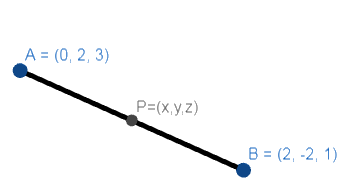Courses
Courses for Kids
Free study material
Free LIVE classes
MoreLIVE
Join Vedantu’s FREE Mastercalss

# Find the locus of the point which is equidistant from the points A(0, 2, 3) and B(2, -2, 1).Verified
362.1k+ views
Hint: Take the point P as (x, y, z) use the distance formula which is,
$\sqrt{{{\left( {{x}_{2}}-{{x}_{1}} \right)}^{2}}+{{\left( {{y}_{2}}-{{y}_{1}} \right)}^{2}}+{{\left( {{z}_{2}}-{{z}_{1}} \right)}^{2}}}$. Then equate PA and PB as they are equidistant and find the result.

Let P assume a point (x, y, z) such that PA distance is always equal to PB distance, as shown in figure below:Then we can represent it as
PA = PB
As the values are the same then if we square their values it will also remain the same.
So we can say,
$P{{A}^{2}}=P{{B}^{2}}$
At first for finding PA we have to use distance formula which is
$\sqrt{{{\left( {{x}_{2}}-{{x}_{1}} \right)}^{2}}+{{\left( {{y}_{2}}-{{y}_{1}} \right)}^{2}}+{{\left( {{z}_{2}}-{{z}_{1}} \right)}^{2}}}$
Where the points are $\left( {{x}_{1}},{{y}_{1}},{{z}_{1}} \right)$ and $\left( {{x}_{2}},{{y}_{2}},{{z}_{2}} \right)$ whose distance is to find out.
So if point are P(x, y, z) and A(0,2,3)
Then,
$PA=\sqrt{{{\left( x-0 \right)}^{2}}+{{\left( y-2 \right)}^{2}}+{{\left( z-3 \right)}^{2}}}$
Now if points are P(x, y, z) and B (2,-2,1).
$PB=\sqrt{{{\left( x-2 \right)}^{2}}+{{\left( y+2 \right)}^{2}}+{{\left( z-1 \right)}^{2}}}$
Now as we know that,
PA = PB
And further we also know,
$P{{A}^{2}}=P{{B}^{2}}$
So we can write it as,
${{(x-0)}^{2}}+{{\left( y-2 \right)}^{2}}+{{\left( z-3 \right)}^{2}}={{\left( x-2 \right)}^{2}}+{{\left( y+1 \right)}^{2}}+{{\left( z-1 \right)}^{2}}$
Now by further expanding by using formula as, ${{\left( a-b \right)}^{2}}={{a}^{2}}+{{b}^{2}}-2ab$ and ${{\left( a+b \right)}^{2}}={{a}^{2}}+{{b}^{2}}+2ab$
We can write is as,
${{x}^{2}}+{{y}^{2}}-4y+4+{{z}^{2}}-6z+9={{x}^{2}}-4x+4+{{y}^{2}}+4y+4+{{z}^{2}}-2z+1$
Now on further simplifying by adding constant we can write it as,
${{x}^{2}}+{{y}^{2}}-4y+{{z}^{2}}-6z+13={{x}^{2}}+{{y}^{2}}-4x+4y+{{z}^{2}}-2z+9$
Now cancelling $\left( {{x}^{2}}+{{y}^{2}}+{{z}^{2}} \right)$ to both the sides of equation we get,
-4y – 6z + 13 = -4x + 4y – 2z + 9
Now adding (4x – 4y + 2z – 9) to both sides of equation we get,
4x – 8y – 4z + 4 =0
Now dividing by ‘4’ throughout the equation we get,
x- 2y – z + 1 = 0
Hence x- 2y – z + 1 = 0 this is the required equation of locus.
Note: In the question we asked to find the locus take is as a variable and try to make an equation or relation with. Also while expanding be careful about any calculation error so that answer comes one go.The locus of a point which is equidistant from two given points is actually the perpendicular bisector of the segment that joins the two points. We can find the locus using this fact as well.
Last updated date: 29th Sep 2023
Total views: 362.1k
Views today: 10.62k